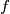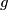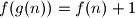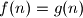### IMO Shortlist 2010 problem A6

Kvaliteta:
Avg: 3,0
Težina:
Avg: 8,0
Suppose that$f$ and$g$ are two functions defined on the set of positive integers and taking positive integer values. Suppose also that the equations$f(g(n)) = f(n) + 1$ and$g(f(n)) = g(n) + 1$ hold for all positive integers. Prove that$f(n) = g(n)$ for all positive integer$n.$

Proposed by Alex Schreiber, Germany
Izvor: Međunarodna matematička olimpijada, shortlist 2010